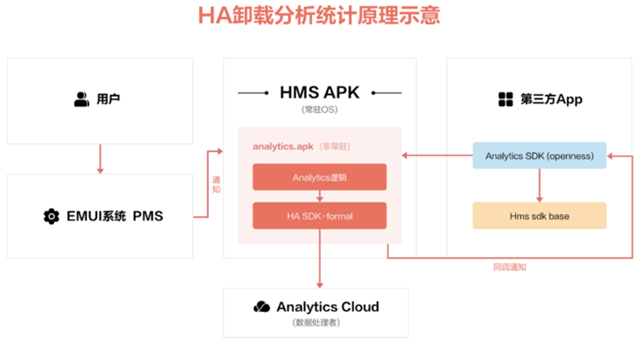# 用户画像TGI指标

## 什么是TGI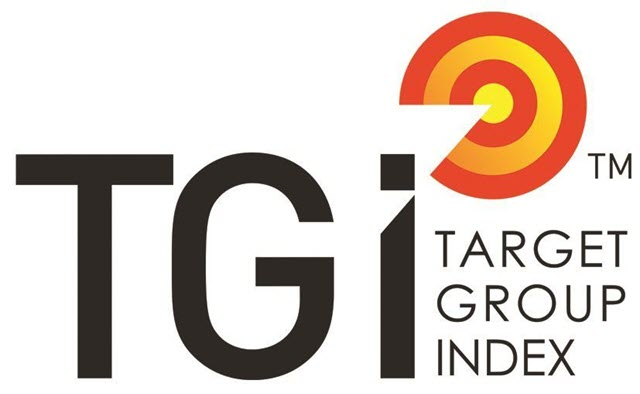TGI指数计算公式 = 目标群体中具有某一特征的群体所占比例 / 总体中具有相同特征的群体所占比例 * 标准数100

• 烧烤店男生TGI=50%/20%*100=250
• 烧烤店女生TGI=50%/80%*100=60

## TGI的使用

TGI指数表明不同特征用户关注的差异情况：

• TGI指数 = 100 表示平均水平，
• TGI指数 > 100，代表该类特征对目标群体的影响要大于平均水平，或者该类群体对某类问题的关注程度高于平均水平
• TGI指数 < 100，代表该类特征对目标群体的影响要小于平均水平，或者该类群体对某类问题的关注程度低于平均水平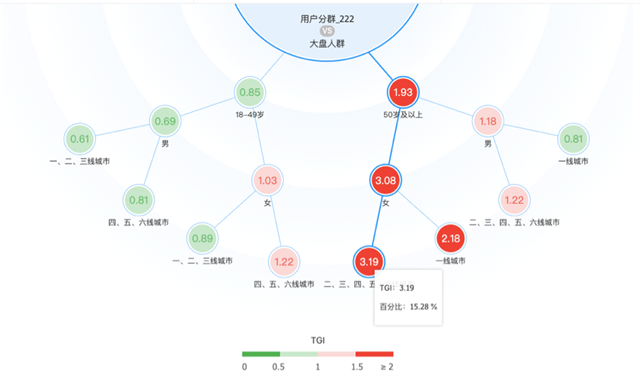## TGI实战

import pandas as pd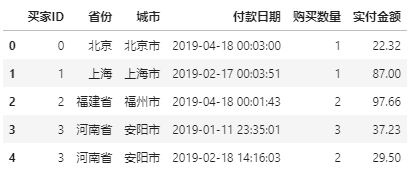df_price = df[['城市', '买家ID', '实付金额', '购买数量']].groupby(['城市', '买家ID']).agg('sum').reset_index()
df_price['客单价'] = df_price['实付金额']/df_price['购买数量']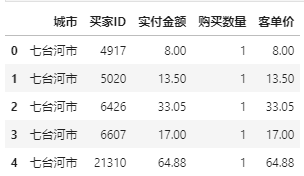df_price_city = df_price[['城市','客单价','买家ID']].groupby(['城市']).agg({'客单价':'mean', '买家ID':'count'}).reset_index().rename(columns={'买家ID':'买家数量'})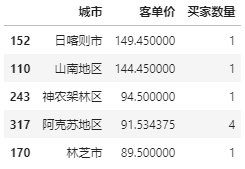df_price_city.loc[df_price_city['买家数量'] > df_price_city['买家数量'].mean(),:].head()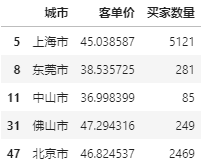df_price['客单价'].hist(bins=16)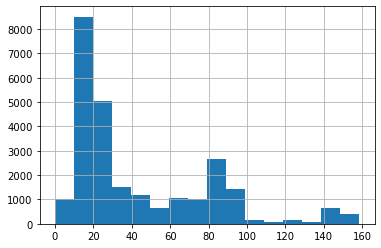threshold = 50
df_price_high = df_price[df_price['客单价']>=threshold][['城市','买家ID']].groupby(['城市']).agg('count').reset_index().reset_index().rename(columns={'买家ID':'买家数量'})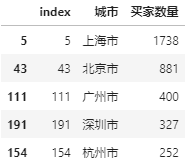df_price['高客单'] = df_price['客单价'].apply(lambda x: 1 if x >=threshold else 0 )
df_price['低客单'] = df_price['客单价'].apply(lambda x: 1 if x < threshold else 0 )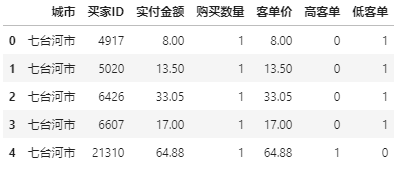df_summary = df_price[['城市','高客单','低客单']].groupby(['城市']).agg('sum').reset_index()
df_summary['总人数'] = df_summary['高客单'] + df_summary['低客单']
df_summary['高客单占比'] = df_summary['高客单'] / df_summary['总人数']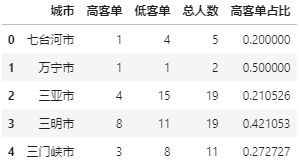total_percentage = df_summary['高客单'].sum() / df_summary['总人数'].sum()
total_percentage


df_summary['高客单TGI指数'] = df_summary['高客单占比'] / total_percentage * 100
df_summary = df_summary.sort_values('高客单TGI指数',ascending = False)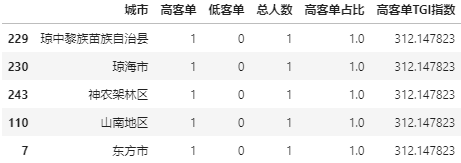df_summary.loc[df_summary['总人数'] > df_summary['总人数'].mean(),:].head()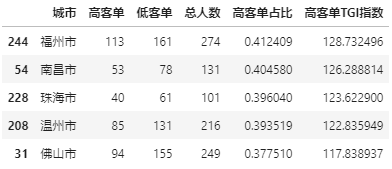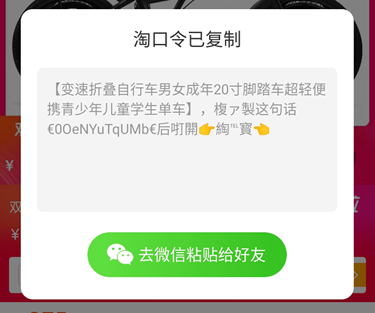##### 淘口令的技术实现##### App“签到/打卡”功能点的思考